Homework Help Question & Answers

Application 16.0 Cost-effective Gunk Control Two plants are emitting a uniformly mixed pollutant ...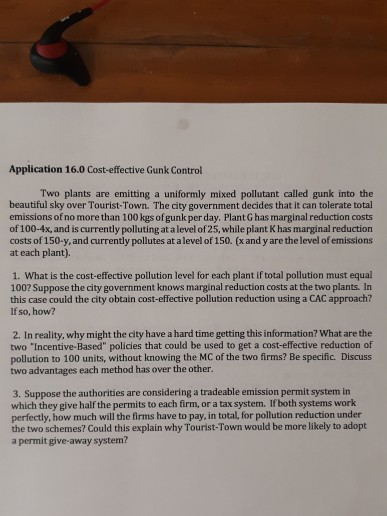Application 16.0 Cost-effective Gunk Control Two plants are emitting a uniformly mixed pollutant called gunk into the beautiful sky over Tourist-Town. The city government decides that it can tolerate total emissions of no more than 100 kgs of gunk per day. Plant G has marginal reduction costs of 100-4x, and is currently polluting at a level of 25, while plant K has marginal reduction costs of 150-y, and currently pollutes at a level of 150. (x and y are the level of emissions at each plant). 1. What is the cost-effective pollution level for each plant if total pollution must equal 100? Suppose the city government knows marginal reduction costs at the two plants. In this case could the city obtain cost-effective pollution reduction using a CAC approach? If so, how? 2. In reality, why might the city have a hard time getting this information? What are the two "Incentive-Based" policies that could be used to get a cost-effective reduction of pollution to 100 units, without knowing the MC of the two firms? Be specific. Discuss two advantages each method has over the other. 3. Suppose the authorities are considering a tradeable emission permit system in which they give half the permits to each firm, or a tax system. If both systems work perfectly, how much will the firms have to pay, in total, for pollution reduction under the two schemes? Could this explain why Tourist-Town would be more likely to adopt a permit give-away system?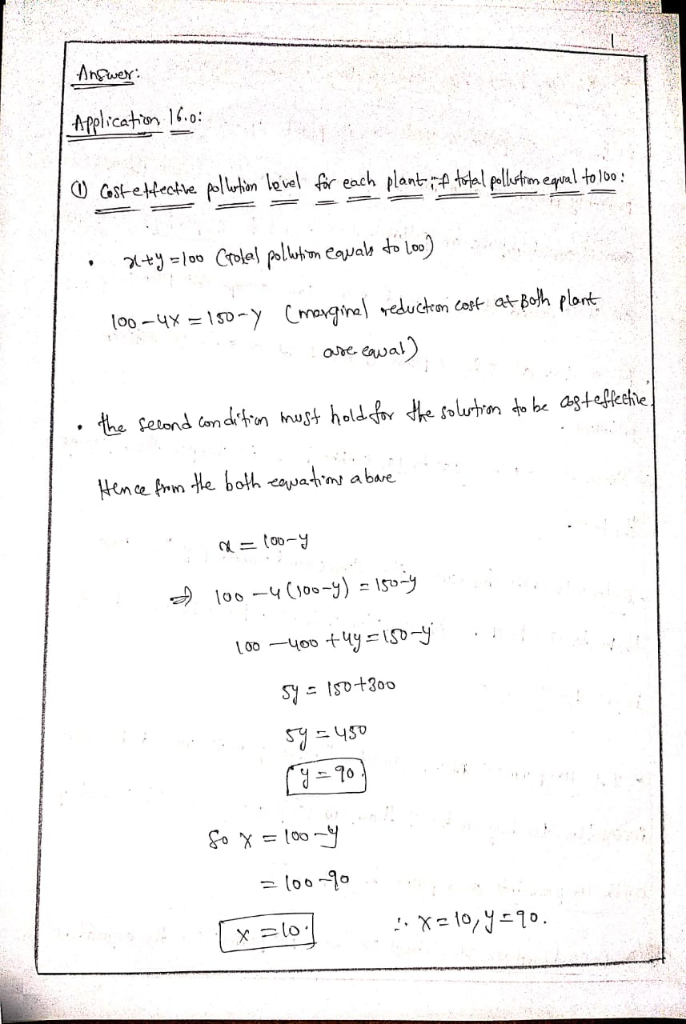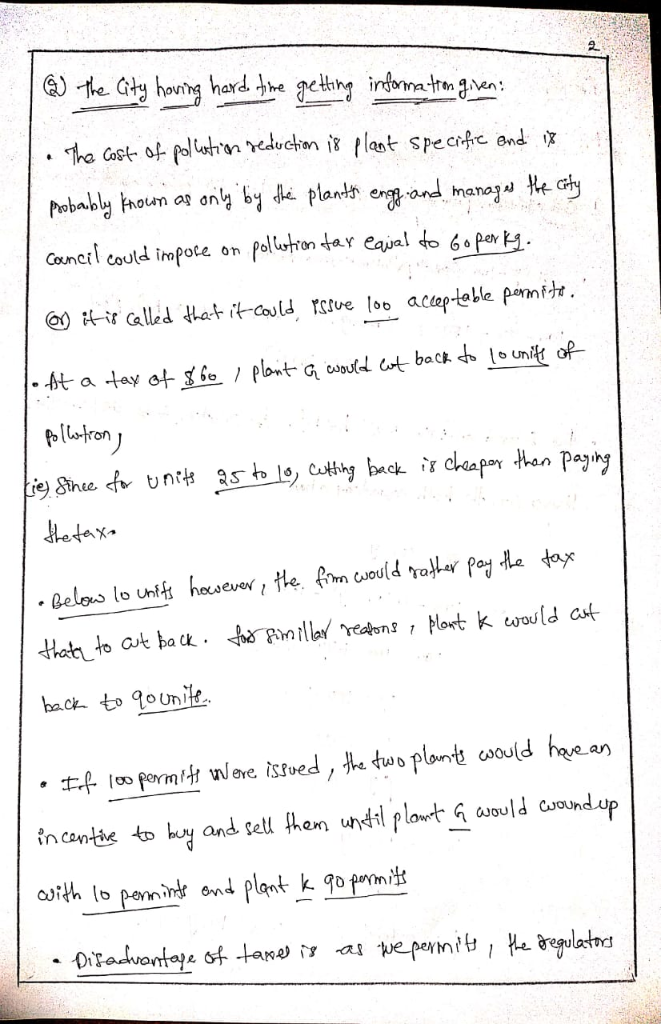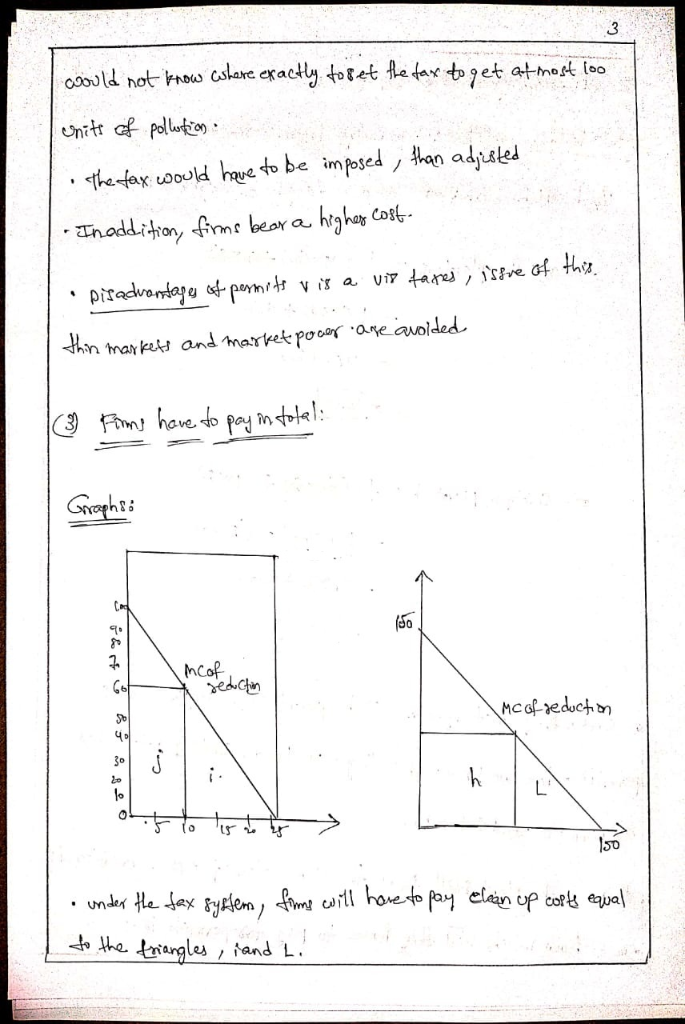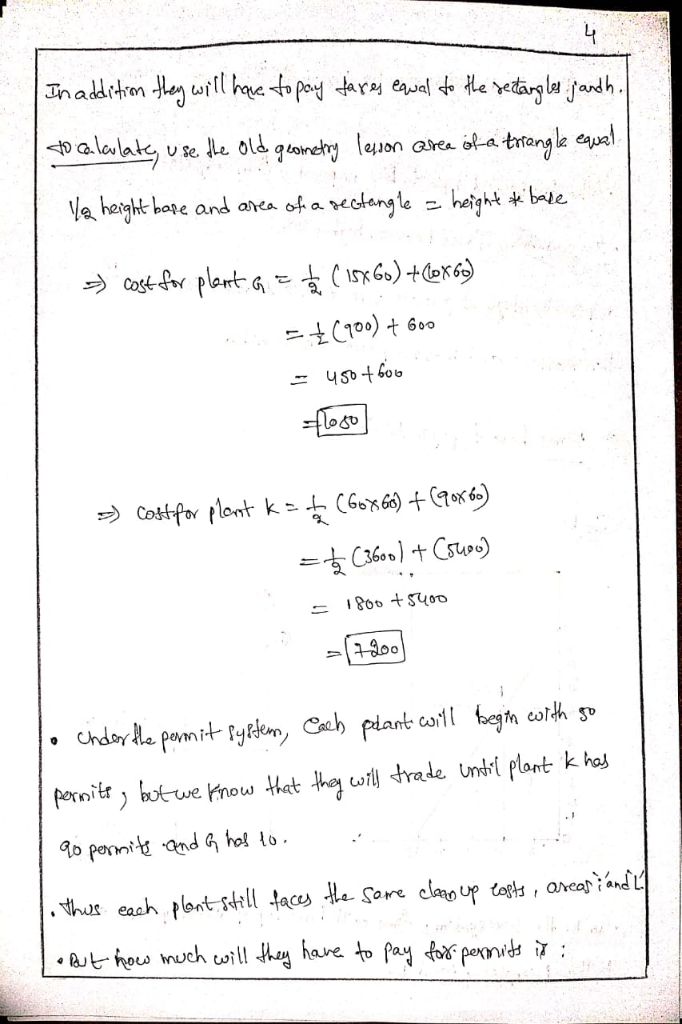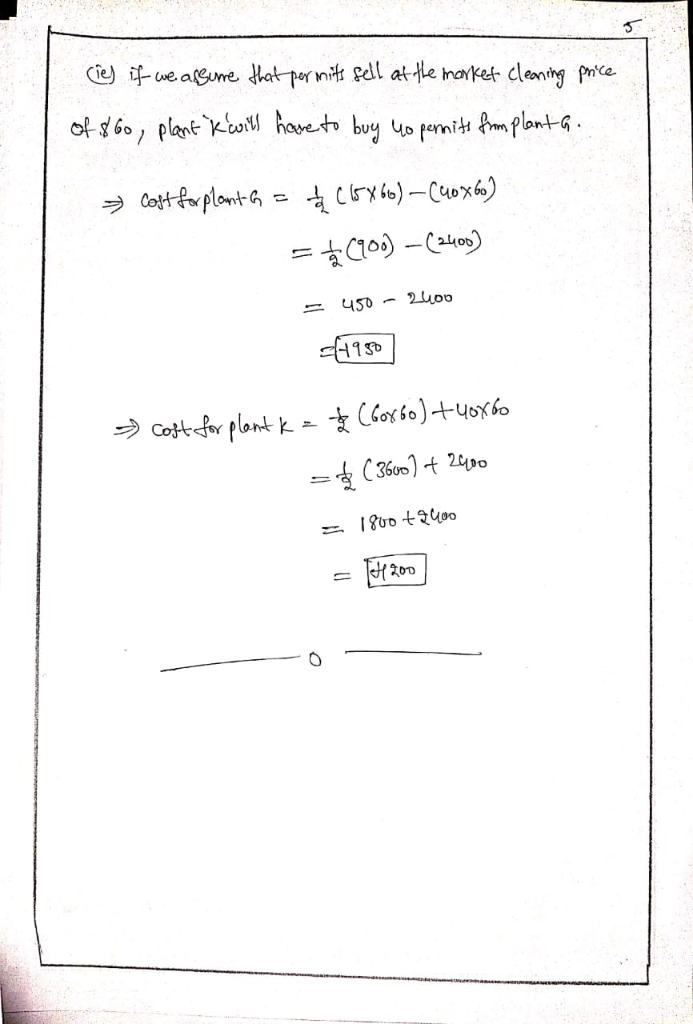Add Answer of: Application 16.0 Cost-effective Gunk Control Two plants are emitting a uniformly mixed pollutant ...
More Homework Help Questions Additional questions in this topic.

• Explain why a 1:3 ratio of green-seeded plants to yellow-seeded plants is the same as a fraction of 1/4 green seeded plants thanks

Need Online Homework Help?

Get FREE EXPERT Answers
WITHIN MINUTES
Related Questions